Disclaimer: This is an example of a student written essay.

Any opinions, findings, conclusions or recommendations expressed in this material are those of the authors and do not necessarily reflect the views of UKEssays.com.

# Wave Function Collapse in Deep Convolutional Generative Adversarial Network

Info: 8731 words (35 pages) Essay
Published: 18th May 2020

The procedural content generation algorithms are a well-known concept in the game industry. Because of their time efficiency, there is more effort put into developing new algorithms. The Wave Function Collapse algorithm developed by Max Gumin is populating the pattern from a small sample. The algorithm gained much popularity because of the variety of the outputs generated from only one input. This paper examines whether the wave function collapse can be trained within a use of Deep Convolutional Generative Adversarial Network to get the output starting with the input specified by the user. This would give additional control over the algorithm and allow to specify the spatial distribution of tiles across the solution space.

Keywords: Wave Function Collapse, Procedural City Generation, Machine Learning, Generative Adversarial Network

List of figures

The numerous benefits have been driving developers to research complex and universal generators that potentially could create an entire virtual world. The complete landscape with architecture, a set of items and complex rules could be the result of one procedural content generation algorithm. Four decades of research still did not bring the solution that can produce the complete game content.  However, there has been a significant advancement in the algorithms that are targeting the specific content category, rather than covering all topics. For example, the L-system has been applied as a road network generator with controllable parameters like road patterns and populace density (Parish and Müller 2011). L-systems and cellular automata are also conventional techniques for procedurally generated behaviour simulating physics like fireworks explosion or background characters movement (Hidalgo, et al. 2008). The phenomenon behind those methods is their simplicity of the logic and complex, unpredictable result. Although the rules are entirely deterministic, the outcomes are widely different due to the initial state. An object following a few simple rules can behave similarly to real-world objects, using at the same time a little computer power. The applications of L-systems and cellular automata range from small scale like plant generation, to a larger one like simple 2D game levels.

If you need assistance with writing your essay, our professional essay writing service is here to help!

The procedural new level generation is one of the most researched applications of the PCG algorithm (Hendrikx, et al. 2011). The challenge of a level generation is to develop the variety between the levels, where each level is not a monotone repetition of the same pattern. The commonly used tools to disrupt the repetitious content are functions that randomise the values. Perlin Noise invented by Ken Perlin (Musgrave, et al. 2003) is a method that generates naturally looking textures that can be utilised by computer graphic designers to create content that resembles the real-life textures, for example, a realistically looking sky (Roden and Parberry 2005). While the functions like Perlin Noise successfully randomise the context, there are not necessarily applicable to the games that require a complex environment with semantics. The techniques applied to generate advance environment are trying to solve the constraint satisfaction problems. The popular PCG methods solving constraining problems are tile-based generators. The tiles are the ingredients of the content, and the constrains specify the relationship between the tiles.

Wave Function Collapse developed by Max Gumin received lately a lot of popularity because of the constrains-based solved that generated a different result from one sample.

Game creators benefit broadly from the use of procedural content generators. The algorithms are commonly applied in the game industry. Despite their popularity, they still face many challenges. Generated content usually looks nonexclusive and requires the purposeful macro-structure. Generated levels vary between each other, but after a few iterations, the player easily can notice the similarities. The lack of progression between the levels decreases the value of the game. The commonly used concept for game progression, are levels evolving towards broader and more complex content together with the progress of the player. To design that kind of progression, the creator needs to get more control of the algorithm. Procedurally generated games are usually not flexible to any additional input beside the set of rules. The meaningful and original content creation needs to have human input. Therefore, the generators should be able to respond to additional instructions added on the top of the set of rules.

This dissertation explores the techniques that could enable an artist to specify the spatial features of the game content and generate the result that satisfies the constrains.

### 1.1.      The aim

This dissertation aims to explore two approaches to generate the Wave Function Collapse result with the additional requirements specified by the designer.  Additionally, to the standard algorithm inputs, there is an image that will indicate the areas of desirable content. In the game context, that could be a river, island or building that follows the desirable distribution. In the presented example, the desirable content ( shown as black area in the input image) corresponds to the non-white cells. Adding the requirements on the top of the Wave Function Collapse, would fully use the benefits of the algorithm, enriching the result with the meaningful macro-structure. Controlling how the generated space looks, enables the artist to create varied complexities of the content and adjust the architecture to the specific level requirements.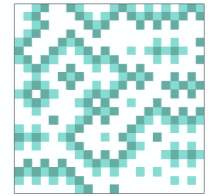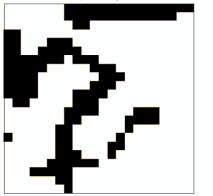Both techniques aim to use a simple image as an indicator where assets should appear. The result should also keep the wave function collapse constrains. The first approach is using Generative Adversarial Networks, and the second approach is modifying the probabilities functions inside the Wave Function Collapse.

Figure 1. Input image.

Figure 2. Result of the Wave Function Collapse.

### 1.2.      The structure of the document

The first chapter introduces the concept and advancements of the procedural content generators and explains the current challenges. The background section describes the Wave Function Collapse algorithm that is the basis for this dissertation. That is followed by relevant research for this topic. The methodology is separated into two different chapters, as this dissertation explores two methods to achieve the aim. Therefore, the third chapter explains the machine learning approach, and the fourth chapter focuses on the second method, which is working with the probabilistic and entropy. The methodology of both approaches is followed by a brief description of results evaluation methods and the results from both methods. The overview and assessment precede the conclusion.

### 2.1.      Introduction to Wave Function Collapse

Wave Function Collapse (WFC) is a procedural content generation algorithm developed by Max Gumin in 2015. The name Wave Function Collapse refers to the quantum mechanics process of changing the superposition of the wave function due to the presence of the observer. High entropy of unobserved state is decreasing proportionally with observing the particles. Once the state is observed, and entropy is equal to zero, the wave function collapses.

The Wave Function Collapse is a tile-based content generator. The input is a sample of the pattern that needs to be populated. The set of tiles is built from the tessellated pattern sample. Each tile has assigned other tiles as possible neighbours. The algorithm is a constraint-based solver which will not allow for any tile connections that do not match the neighbour settings.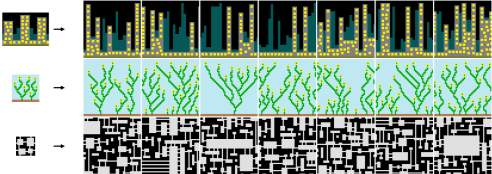The first application of the WFC was 2D patterns generated by the author itself (Gumin 2015). Examples were presenting the applications of the pattern in different sceneries like city elevations, brick layouts or abstract patterns.

Figure 3. 2D patterns generated with Wave Function Collapse by Max Gumin.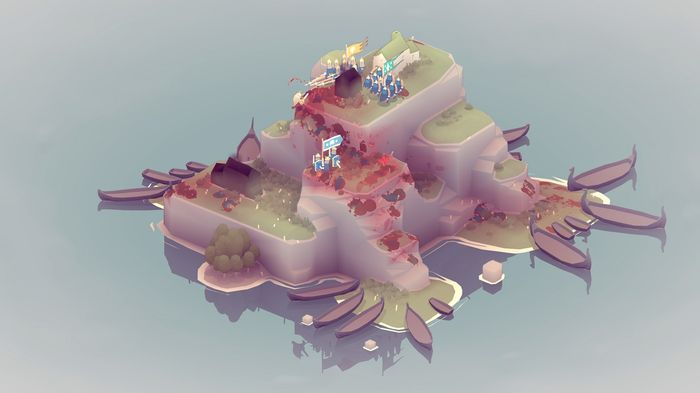WFC can be applied both to two- and three-dimensional space. In a 2D, one tile can be a pixel, image or a 2D surface. In a 3D, tile can be a voxel or a 3D model. Marian Kleineberg adapted WFC to create an infinite city assembled from 3D blocks like bridges, buildings and streets. The content continues to generate further in any direction indisposing the user walking through the city to reach the end of the virtual city (Kleineberg, 2018). One of the first games generated with the use of Wave Function Collapse is a Proc Skater 2016 (Parker, Jones and Morante 2016). It is a skateboarding game in which a player can enjoy numerous procedurally generated skate parks and save the favourite configuration. A real-time strategy video game Bad North (Stålberg and Meredith 2018)uses WFC in three-dimensional space to generate islands which are game levels. This publication attracted much attention not only with the use of WFC, but also with the outstanding aesthetics.

Figure 4. Island generated with Wave Function Collapse in the video game Bad North.

Since the first publication of the algorithm, the interest coming from both hobbyists and professionals led to numerous applications and modifications of the WFC. It also became a topic of academic research in the field of game design and procedural content generation.

### 2.2.      Wave Function Collapse explained

Wave Function Collapse is the starting point of this dissertation. The algorithm was recreated based on the first academic publication concerning the WFC (Karth and Smith 2017). Karth and Smith describe the history and applications of the method followed by the explanation of each step with the pseudocode allowing to understand and recreate the algorithm. Understanding the algorithm is essential to follow the further part of this dissertation. Therefore, this section will be an explanation of each step of the Wave Function Collapse presented on the example that is also an input pattern for the experiments.

Input pattern sample

The input pattern is a sample of the content to be populated. It could be a bitmap where one cell is one pixel or a grid of elements where one cell is one 2D or 3D asset. The image used for this dissertation is a simple 2D image build from three types of components. The logic of the WFC is the same for every level of complexity. However, a more straightforward pattern is time-efficient. The pattern sample is a grid of a five by five size. Three types of components are filling the grid. In this particular example, the shape of the components is the same, and the difference is only in colour. For the clear referencing in this document, each component (also called a cell) has its letter code that will be used in the further parts of this dissertation.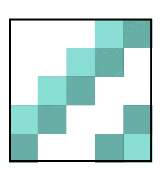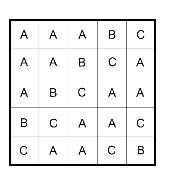Figure 6. Pattern sample encoded as letters.

Figure 5. Pattern sample.

Library of patterns from pattern sample

Gumin proposes two models for the pattern (also called tile) library generation: the simple tiled model and the overlapping model. The first method is tessellating the input pattern into the grid of size NxN. Commonly used size is 2 or 3. One cell in the grid becomes one pattern. The overlapping model extracts all possible NxN patterns. That means that a library generated with the overlapping method will have more members than the one generated by a simple tiled model. For both methods, the pattern can be reflected and rotated in order to build a more extensive library of patterns. The simple tiled model is more computationally efficient in the later stages of the algorithm. The overlapping model generates a more extensive library of patterns which results in the more diversified output. The impact of choosing one model or another is especially influent when dealing with straightforward patterns. For the intricate input patterns, the result diversifies with both models. With the simple pattern sample, the simple tiles model will generate a small library of patterns, and the outcome of the Wave Function Collapse can be less attractive.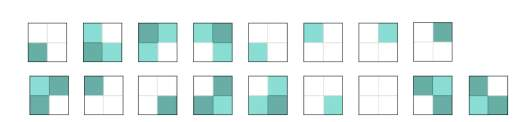Because of the simplicity of the input pattern sample used in this work, the library of tiles is generated using the overlapping model.

Figure 7. Library of patterns generated with overlapping model and rotation.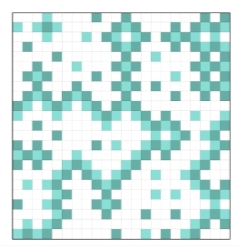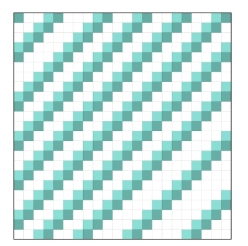Figure 9. WFC result built from the overlapping model with rotation library.

Figure 8. WFC result built from the simple tiled model patterns library.

Probability

Each tile from the generated library has an assigned weight. The weight corresponds to the probability of this pattern appearing in the solution. The weight of the pattern is the sum of weights of the components that build this pattern. The component’s weight is representing the percentage of the sample image that this component is filling. For example, the sample pattern presented in the previous section (Figure 3) has 52 % of the A components, 20% of the B components and 28% of C.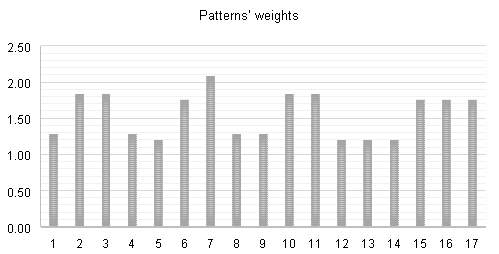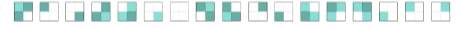Table 1. Weights of the patterns.

Overlapping neighbours

The next step is to assign a set of possible neighbours that can appear next to the pattern. For the overlapping model, each tile has (2( N – 1) + 1)2  offsets to consider.

Entropy

Each cell in the space that needs to be solved has its entropy. The entropy is proportional to the number of patterns that will satisfy the constraints in this location.  As the more patterns appear in the solution, the entropy decreases. Similarly to the quantum mechanic’s concept of the information, the entropy can increase but never decrease, and the entropy of pure state is equal to zero (Nielsn and Chuang 2000). The values are calculated from Shannon’s Entropy (Shannon 1948) equation: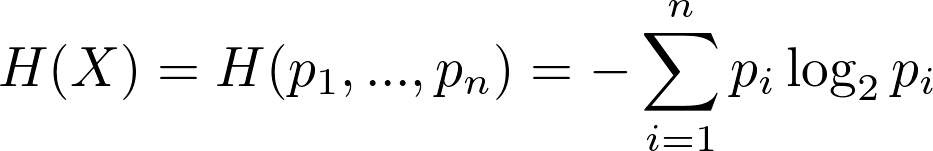Where pi is the weight of the pattern. That means that a cell with only a few patterns possible will have smaller entropy than the cell where many tiles will satisfy the constraints.

Observation

Once the generated set of tiles has assigned a list of possible neighbours and weight, the next step is to select the first pattern to collapse. At this point, the entropy is equally high at every cell. Therefore the first location is usually set to random or specified by the user. With every next iteration, the selected cell for the new pattern has the lowest entropy. Once the location is defined, the next step is to pick which pattern will appear in this place. The patterns with higher weights have a higher probability of being selected.

sum = sum all weights from possible_patterns

random = get random number from zero to sum

current_sum = 0

go through every possible pattern from possible_patterns:

current_sum = current_sum + weight of current pattern

if (current_sum) > random:

return this pattern as selected

Propagation

Once to the selected location, there is selected a pattern. The next step is to update the entropies. The entropy of the selected location drops to zero, because it is an observed state. Then, for each of the overlapping positions, the entropy needs to be recalculated, as the patterns that cannot be neighbours to the newly selected pattern needs to be blocked. Therefore the entropy of those locations also decreases.

The observation and propagation steps repeat until the whole space is not solved.

With every iteration, more patterns get blocked, and the entropy decreases. It may happen, that in a location where there is still no pattern assigned, the number of possible patterns will be equal to zero. This circumstance is called a contradiction. In Gumin’s version of Wave Function Collapse, if the process gets to this point, it resets and starts from the beginning. In the later adaptations of the algorithm, with the backtracking the step that causes contradiction can be ‘erased’ so different pattern can be assigned. The backtracking does not solve the problem of contradictions completely; however, in many cases, it reduces it significantly. Wave Function Collapse has known scalability limitations (Scurti and Verbrugge 2018)  and more complex input patterns, and larger output pattern rapidly increases the computing time mainly because of the growing number of contradictions.

The describes guidelines above are the underlying implementation of the Wave Function Collapse with one additional element – backtracking. However, the algorithm is often modified to adjust the functionality to a specific problem.

### 2.3.      Machine learning and Wave Function Collapse

With the growing interest of the PCG algorithm such as Wave Function Collapse researchers are exploring new methods for creating valuable content with minimal human assistance. Procedural Content Generation through Machine Learning (PCGML) is a novel concept of a game content creation using machine learning models trained on existing content (Summerville, et al. 2017). The recent research in PCGML concentrates on reproducing the game assets to provide the numerous variations of the virtual environment, trained on the previous examples. However, the more design endeavour invested in delivering high-quality training data, the lower the payoff of applying the PCGML in the first place. Karth and Smith propose implementation of discriminative model into the modified version of Wave Function Collapse, where the model learns to assess whether a generated content is acceptable. Both negative and positive examples of the generated patterns feed the discriminator paired with WFC examples (Karth and Smith 2018). Through incrementation of the inputs ( Gumin’s WFC allows for one input) artist feed the algorithm with examples of patterns that did not appear in the sample pattern but would be a positive variety to the original pattern. This technique requires more human contribution that original WFC model, however, it encourages the artist to make changes in the pattern by adding new features rather than recreating the new sample pattern.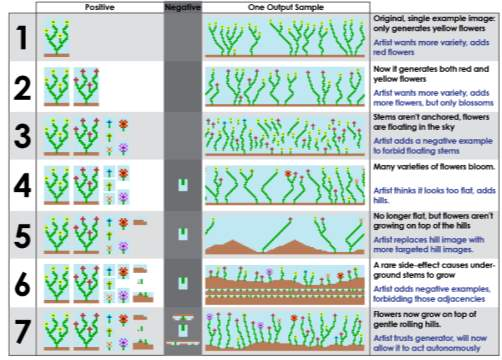Figure 10. Karth and Smith’s example of the WFC combined with the discriminator fed by positive and negative examples.

### 2.4.      Controllable procedural content generation

I found a paper “Controllable Procedural Content Generation  via Constrained Multi-Dimensional Markov Chain Sampling” so it is not exactly wave function collapse, but maybe it is relevant, so maybe I will shorty write about it.

### 2.5.      Algorithmic complexity

Not sure if this is still relevant, but maybe short paragraph about that the problem is combinatorial, therefore it is very complex and contrained etc, so it is hard to make it flexible.

### 3.1.      Introduction

The first proposal to generate Wave Function Collapse result that responds to the additional input image, is to use a machine learning model to train the model on the collected WFC results data. The model should be supplied with both the input image and the right WFC outcome, in order to train the relationship between each other.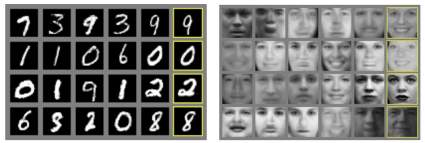A Generative Adversarial Networks (GAN) is a machine learning model that concurrently train two neural networks: a generative and discriminative one (Goodfellow, et al. 2014). A generative model (called Generator) learns the data distribution and attempts to produce a data resembling the training dataset. Simultaneously, a discriminative model (called Discriminator) learns to distinguish whether the data comes from the generator or the actual dataset. GAN, described as the most exciting idea in the last decade of machine learning (LeCun 2016) received much attention from research, which led to multiple variations of the model. The image-to-image translation, additionally to the mapping from the input image to the output image, also learns a loss function to train this mapping (Isola, et al. 2016).

Figure 11. Two examples presented in (Goodfellow, et al. 2014). The right column shows the results of the generated image after the training.

• Describe the architecture of image-to-image translation

### 3.2.      Dataset preparation

The training, testing and validation data for the image-to-image translation are the results of the Wave Function Collapse outcomes. The dataset contains 1200 pairs for training, 400 for testing and validation. Image-to-image translation can produce satisfactory results with the small data size of around 400 images. However, the type of images trained in this example varies from the commonly applied dataset. Each image in the dataset is a pair of the input image and the desirable WFC outcome ( see Figure 1 and 2). The black and white input image is a processed Wave Function Collapse result image. The first iteration removes components B and C that are surrounded only by white A components and replace them with A. Then, the gaussian blur masks the details of the image, and extract the macro-structure of the pattern. The last step is a mask that converts the pixels of the brightness below 0,7 to black, and brighter than this threshold pixel to white.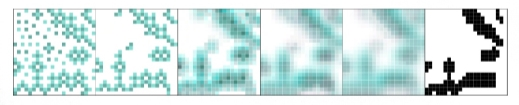### 3.3.      Image encoding

The images usually trained in GAN model are photographies or drawings. The changing values of the pixels next to each are the gradients that usually mean this is an edge of the shadow. In the case of WFC output, the pixels have a different type of relationship and should not be considered as a gradient. Therefore, to differentiate the values, rather than assigning values as RGB or brightness values, each pixel is converted to the one state. Explain one-hot encoding.

The pattern has three different values. Each value has its combination of one 1 and zeros that is unique for this colour.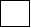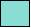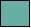[ 1, 0, 0 ]   [ 0, 1, 0 ]   [ 0, 0, 1 ]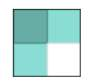The one-hot encoded pattern is an array of four corresponding to the cell colours values:

[  [ 0, 0, 1 ] ,   [ 0, 1, 0 ],

[ 0, 1, 0 ] ,   [ 1, 0, 0 ]  ]

The colour value encoding, follows the information about the cell’s neighbours. That means that six values represent one cell: the first three  are colour codes, and the last three are the sums of

### 3.4.      Image-to-image translation

• The pix2pix model is using the TensorFlow libraries
• Generator architecture (especially downsampling, upsampling, and activation functions)
• Discriminator architecture (same as in generator)
• training
1. Method II: Probability and entropy

### 4.1.      Introduction

The second approach aims to generate Wave Function Collapse pattern that reflects the geometry from the input image, following the steps of the original algorithm. As opposed to the first method, this proposal focuses on fully satisfying the constrains. Therefore, the core logic remains the same, and the focus is on the modifications of weights of the patterns and the entropies. By increasing the weights of desirable components in the areas corresponding to the black pixels in the input image, the probability of WFC choosing those patterns increases. This approach presents three different functions for weight recalculation, tested on the set of different values.

### 4.2.      Input image

The input to the algorithm is the pattern sample (the standard input to the Wave Function Collapse, see Figure x) and the second input which is black and white image (see Figure x).  Input image describes a macro-structure of the desirable WFC output where black pixels corresponds to the cells B and C (darker colours).

Our academic experts are ready and waiting to assist with any writing project you may have. From simple essay plans, through to full dissertations, you can guarantee we have a service perfectly matched to your needs.

### 4.3.      Linear mapping of the probabilities

The first approach is heavily relying on the original weights calculated from the pattern sample. The values of each cell (A, B and C) exchanges between each other, so the higher weight, previously assigned to white cell A, becomes a weight of the cell C (the darker one). Tile B has medium brightness, and the weight stays the same.

Because the structure of the tiles is different, and while in the original set the highest tile was built from 4 white components, with the new weights his highest value cannot be obtained because the patterns are built in a way that there is no cell with four dark green cells. To keep the same domain of the values, each new pattern weight is mapped into the original weight domain.

The initial step to recalculate the weights was to reverse the existing proportions. The highest weight initially assigned to white cell (0.52) now is assigned to the dark green cell. The middleweight (0.28) is for light green cell and the lowest (0.20) for white cell.

In the chart (see Table 1), the method I are values of reversing the weights, the method II presents the same weights mapped into original pattern weight domain.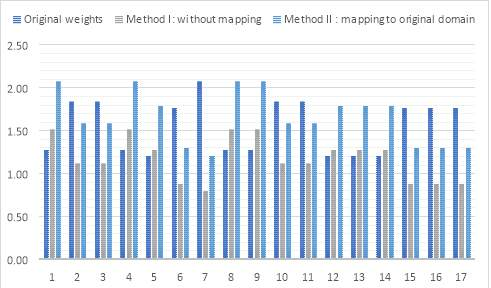Table 2 Comparison of the original weights of patterns, and the modified according to the method 1.

Because the domain stays the same, and the proportions of the weights corresponds to the original values, this will result in similarly random output as the one generated with weights calculated from input image.

The constraints in the algorithm are overwhelming the changed weights. Even giving more probability to the dark cells, the structure of the input image and the dominance of white cells influence the type of the set of tiles that is generated in the first place. Therefore, changing the weights within the same domain, is not effective because the generated set of tiles and natural proportions of input image predefine the pattern.

Because of that reason, the weights have been recalculated enforcing the weights of the green cells to measure what is the result based on those weights. The tested set of weights increase iteratively the light green and dark green values and decrease value .

 weights    0.52/0.20/0.28 0.52 0.2 0.28 0.40/0.30/0.30 40 30 30 0.30/0.25/0.35 30 35 35 0.20/0.40/0.40 20 40 40 0.10/0.45/0.45 10 45 45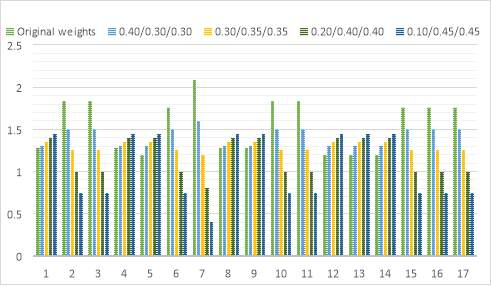Table 3 The patterns weights based on different cell colour weight values

#### 3.3.1. Exponential growth

The second approach is enforcing the green cell exponentially, not linearly. To force the algorithm to put the darker cells, the weights will be calculated exponentially. That means that the difference between white pattern, and dark pattern will be significantly different.

if the sum of cells  and  in the pattern is:

zero:

return pattern_weight

one:

return pattern_weight2

two:

return pattern_weight 3

three:

return pattern_weight 4

The same sets of different weights calculated with exponential growth rewarding tiles with less white space, results in higher domain of output values.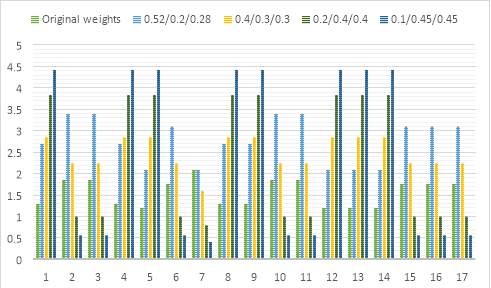#### 4.3.1.     Enforcing the patterns building desirable content

The third approach looking closely to the structure of the regions in the solution pattern that are filled with colourful cells. From the data set that was prepared for the machine learning approach, the occurrence of each pattern in the extracted area is the value feeding this approach.

1. run wave function collapse
2. process the image
3. get the dark are of the image
4. calculate pattern occurrence in the dark area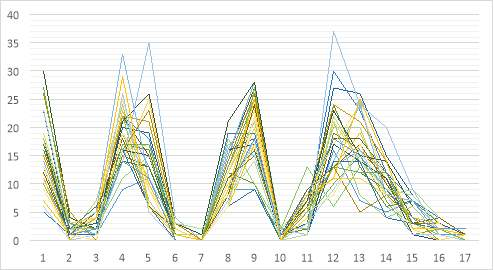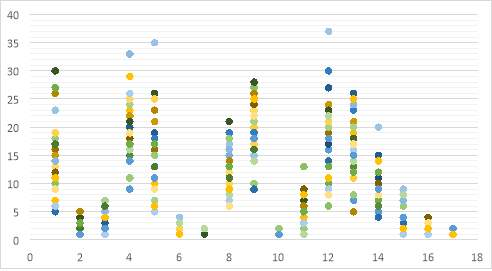Table 4 Pattern occurrence in the areas that are marked black in the input image. The chart visualises 30 iterations, which clearly shows the tendency of which patterns are components of successful run.

The results of the pattern occurrence show a clear selection preference. The average of one hundred iterations is the multiplier for the pattern weights values.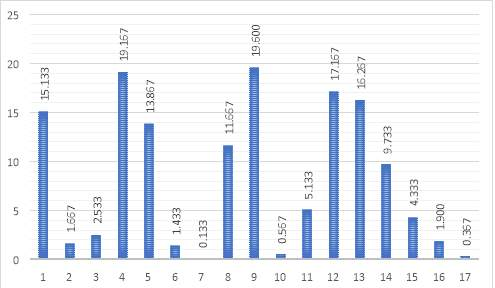Table 5 The average number of pattern occurrence in the dark areas of the image.

### 4.4.      Entropy

The high entropy at the beginning of the solving the output pattern is gradually decreasing in pair with succeeding patterns collapsing. After each collapsed pattern, the location with the lowest entropy without assigned pattern is selected as a next location to be solved. Because of the constraints and the entropy order, once the algorithm naturally gets to the areas marked as black, it is usually already party determined by the collapsed patterns, which pattern can be placed to keep the constraints satisfied. To increase the change of solving the space with desirable patterns, the cells corresponding to the black space have priority and are solved first. The benefits of that are highly dependent on the complexity of the input image. For the more complex shapes, the forced order of collapsing patterns more often results in the contradictions.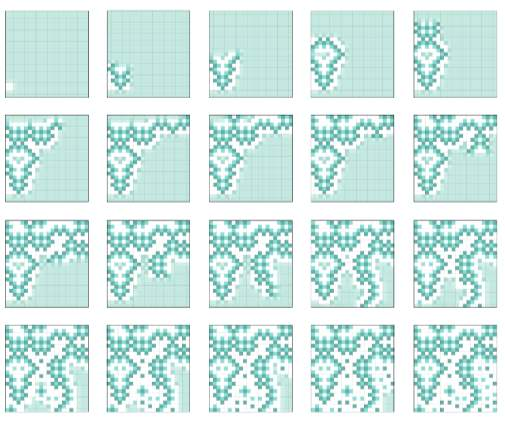Figure 16 The process of content generation with wave function collapse, following the mimimun entropy heuristics.The natural order of collapsing patterns follows is dependant on the structure of the built space. The algorithm first solves the areas with non-white space. Therefore those tiles are the most constrained. With the input image, algorithm still prioritizes lowest entropy locations within the black image’s area, after that it does the same with the areas outside.

### 4.5.      Result measurement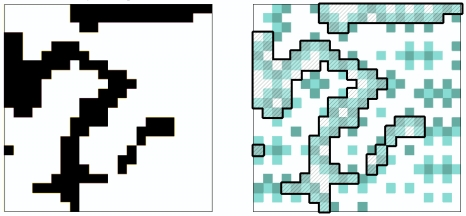.

For 129 cells:

• 36 x  (white)
• 49 x  (light green)
• 44 x  (dark green)

For this example, the success rate is: (49 + 44)/129 = 0.72. Considering that the maximum that can be obtained is 0.75, this model is 96% accurate.

Probability

The probability of appearing pattern in the space partially results of the pattern’s weight, partially due to the random factor. This randomness is the only element of the whole algorithm which is not deterministic. With each iteration, once the next cell to solve is calculated, all the patterns that could appear in this cell are passed to the function that will decide which pattern will be assigned. Modification of the legal patterns would cause the incompliance with constrains, therefore only probabilities are modified. The probability directly results of the weights of the pattern. Therefore, when WFC is running with the image, the weights are recalculated based on the image values.

The original weights are: 0.52 for the white cell, 0.20 for the light green cell, and 0.28 for the

The results are presented based on the simple shaped input image as it enables them to verify whether the proposed solution has successful results. The methods of result evaluation are adjusted to each technique.

### 5.1.      Methods of evaluation

5.1.1.      Analysis of the results in the areas that user-specified as desirable for specific pattern to appear

This method is analysing the cells used to build the area marked as black in the input image. The term success rate represents the proportion of the non-white values to the sum of all cells in that area.

The method I: Generative Adversarial Networks

Rescale the result, run the same operation as on the method II files.

Method II: Modification of probability and entropy

1. Linear mapping

Chart – (data exported)

2. Exponential growth

Chart – (data exported)

3. Enforcing patterns building desirable context

Results:

The chart visalising both methods together, and after that summary:

The results of the image-to-image translation usually gives very high rates. Because the neighbour relatioship of the wfc is not introduced to the algorithm, the input image has much higher priority comparing to the second approach.

The resutls of the second approach is changing according to the method calculating the weights. As predicted, the rate is increasing, when the weighs of pattrern B and C gets higher, while weakening the pattern A. The exponential weight calculation is reaching up to xx success rate. The method III gave the highest results with the xx success rate. The understanding of the structure of the pattern rather than manipulation of the single components gave more promising results.

Evaluate also white spaces

5.1.2.      Image distance (vectors, distances etc etc):

• Image distance
• Colour, brightness ???
• Process image (like to pix2pix) and then check image distance
1.   Constrains check -WORKS

This evaluation method checks whether the content generated using Image-to-Image translation keeps the constrains of the wave function collapse model. All dataset had been tested  and results can be read in the table 12.

PUT HERE A CHART WITH RESULTS

The proportion of patterns from the library to the new illegal patterns decreases with the progress of the neural networks. This is howerer only tool to partly evaluate the result. With the first new epochs, all the patterns exists in the library, however the picture is very limited and it is builded mainly from the empty patterns. In the early epochs 100% of patterns is corrent, the number drops to the 66 % in the latest epochs.

5.1.4.      Pattern occurrence in GAN – WORKS

The pattern occurrence calculates how many times each pattern from generated library of patterns appears in the GAN result. This gives better understanding of the table with pattern constrains and explains the phenomena of high constrain kept rate even in the early epochs.

CHART HERE WITH PATTERN OCCURRENCE

### 5.2.      Comparison of results

Maybe some chart that can fit all the results? Or only summary?

Maybe summary of method I and then summary of method II and compare them?

• Gachagan, Anthony , Chigozie Enyinna Nwankpa, Winifred Ijomah, and Stephen Marshall. 2018. “Activation Functions: Comparison of Trends in Practice and Research for Deep Learning.”
• Goodfellow, Ian, Jean Pouget-Abadie, Mehdi Mirza, Bing Xu, David Warde-Farley, Sherjil Ozair, Aaron Courville, and Yoshua Bengio. 2014. “Generative Adversarial Nets.” Advances in Neural Information Processing Systems 27 .
• Isola, Phillip , Jun-Yan Zhu, Tinghui Zhou, and Alexei A. Efros. 2016. “Image-to-Image Translation with Conditional Adversarial Networks.” IEEE Conference on Computer Vision and Pattern Recognition (CVPR).
• Karth, Isaac, and Adam M. Smith. 2018. “Addressing the Fundamental Tension of PCGML with Discriminative Learning.” Procedural Content Generation. San Luis Obispo.
• —. 2017. “WaveFunctionCollapse is Constraint Solving in the Wild.” Proceedings of the 12th International Conference on the Foundations of Digital Games. Hyannis, Massachusetts, USA: Adventure Works Press.
• Kleineberg, Marian. 2018. Github. 15 July. Accessed 2019. https://github.com/marian42/wavefunctioncollapse.
• Scurti, Hugo, and Clark Verbrugge. 2018. “Generating Paths with WFC.” Proceedings of the Fourteenth Artificial Intelligence and Interactive Digital Entertainment Conference .

View all

## DMCA / Removal Request

If you are the original writer of this essay and no longer wish to have your work published on UKEssays.com then please:

Related Services

Related Lectures